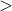# 7.7 The conditional operator

The conditional operator provides a compact means of inserting conditional expressions. Following the syntax of C, it takes three arguments and is written as a ? b : c. The first argument, a is a truth criterion to be tested. If the criterion is true, then the operator returns its second argument b as its output. Otherwise, the function’s third argument c is returned.

pyxplot> f(x) = (x0)?x:0
pyxplot> print "%s %s %s %s %s"%(f(-2),f(-1),f(0),f(1),f(2))
0 0 0 1 2
pyxplot> x = 2
pyxplot> print "x is %s"%(x0)?"positive":"negative"
positive